Next: Distribution functions Up: Monte-Carlo methods Previous: Introduction

## Random numbers

No numerical algorithm can generate a truly random sequence of numbers, However, there exist algorithms which generate repeating sequences of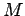(say) integers which are, to a fairly good approximation, randomly distributed in the range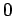to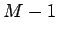. Here,is a (hopefully) large integer. This type of sequence is termed psuedo-random.

The most well-known algorithm for generating psuedo-random sequences of integers is the so-called linear congruental method. The formula linking theth and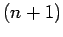th integers in the sequence is(312)

where,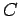, andare positive integer constants. The first number in the sequence, the so-called seed'' value, is selected by the user.

Consider an example case in which,, and. A typical sequence of numbers generated by formula (312) is(313)

Evidently, the above choice of values for,, andis not a particularly good one, since the sequence repeats after only four iterations. However, if,, andare properly chosen then the sequence is of maximal length (i.e., of length), and approximately randomly distributed in the rangeto.

The function listed below is an implementation of the linear congruental method.

// random.cpp

// Linear congruential psuedo-random number generator.
// Generates psuedo-random sequence of integers in
//  range 0 .. RANDMAX.

#define RANDMAX 6074  // RANDMAX = M - 1

int random (int seed = 0)
{
static int next = 1;
static int A = 106;
static int C = 1283;
static int M = 6075;

if (seed) next = seed;
next = next * A + C;
return next % M;
}

The keyword static in front of a local variable declaration indicates that the program should preserve the value of that variable between function calls. In other words, if the static variable next has the value 999 on exit from function random then the next time this function is called next will have exactly the same value. Note that the values of non-static local variables are not preserved between function calls. The = 0 in the first line of function random is a default value for the argument seed. In fact, random can be called in one of two ways. Firstly, random can be called with no argument: i.e., random (): in which case, seed is given the default value 0. Secondly, random can be called with an integer argument: i.e., random (n): in which case, the value of seed is set to n. The first way of calling random just returns the next integer in the psuedo-random sequence. The second way seeds the sequence with the value n (i.e.,is set to n), and then returns the next integer in the sequence (i.e.,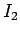). Note that the function prototype for random takes the form int random (int = 0): the = 0 indicates that the argument is optional.

The above function returns a pseudo-random integer in the rangeto RANDMAX (where RANDMAX takes the value). In order to obtain a random variable, uniformly distributed in the range 0 to 1, we would write

x = double (random ()) / double (RANDMAX);

Now ifis truly random then there should be no correlation between successive values of. Thus, a good way of testing our random number generator is to plotversus(wherecorresponds to the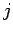th number in the psuedo-random sequence) for many different values of. For a good random number generator, the plotted points should densely fill the unit square. Moreover, there should be no discernible pattern in the distribution of points.Figure 91 shows a correlation plot for the first 10000-pairs generated using a linear congruental psuedo-random number generator characterized by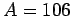,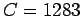, and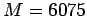. It can be seen that this is a poor choice of values for,, and, since the pseudo-random sequence repeats after a few iterations, yieldingvalues which do not densely fill the interval 0 to 1.Figure 92 shows a correlation plot for the first 10000-pairs generated using a linear congruental psuedo-random number generator characterized by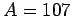,, and. It can be seen that this is a far better choice of values for,, and, since the pseudo-random sequence is of maximal length, yieldingvalues which are fairly evenly distributed in the range 0 to 1. However, if we look carefully at Fig. 92, we can see that there is a slight tendency for the dots to line up in the horizontal and vertical directions. This indicates that theare not quite randomly distributed: i.e., there is some correlation between successivevalues. The problem is thatis too low: i.e., there is not a sufficiently wide selection of differentvalues in the interval 0 to 1.Figure 93 shows a correlation plot for the first 10000-pairs generated using a linear congruental psuedo-random number generator characterized by,, and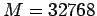. The clumping of points in this figure indicates that theare again not quite randomly distributed. This time the problem is integer overflow: i.e., the values ofandare sufficiently large that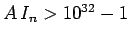for many integers in the pseudo-random sequence. Thus, the algorithm (312) is not being executed correctly.

Integer overflow can be overcome using Schrange's algorithm. Ifthen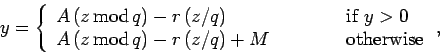(314)

whereand. The so-called Park and Miller method for generating a pseudo-random sequence corresponds to a linear congruental method characterized by the values,, and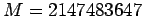. The function listed below implements this method, using Schrange's algorithm to avoid integer overflow.
// random.cpp

// Park and Miller's psuedo-random number generator.

#define RANDMAX 2147483646 // RANDMAX = M - 1

int random (int seed = 0)
{
static int next = 1;
static int A = 16807;
static int M = 2147483647;   // 2^31 - 1
static int q = 127773;       // M / A
static int r = 2836;         // M % A

if (seed) next = seed;
next = A * (next % q) - r * (next / q);
if (next < 0) next += M;
return next;
}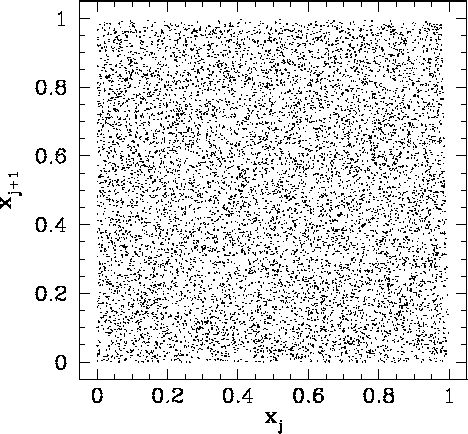Figure 94 shows a correlation plot for the first 10000-pairs generated using Park & Miller's method. We can now see no pattern whatsoever in the plotted points. This indicates that theare indeed randomly distributed in the range 0 to 1. From now on, we shall use Park & Miller's method to generate all the psuedo-random numbers needed in our investigation of Monte-Carlo methods.Next: Distribution functions Up: Monte-Carlo methods Previous: Introduction
Richard Fitzpatrick 2006-03-29